# Consider the matrix A and the reduced row echelon form of A. 1 -2 -5 [1...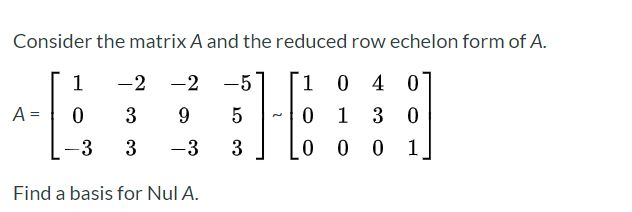Consider the matrix A and the reduced row echelon form of A. 1 -2 -5 [1 -2 9 0 4 0 1 3 0 A= 0 3 5 0 -3 3 -3 3 0 0 0 1 Find a basis for Nul A.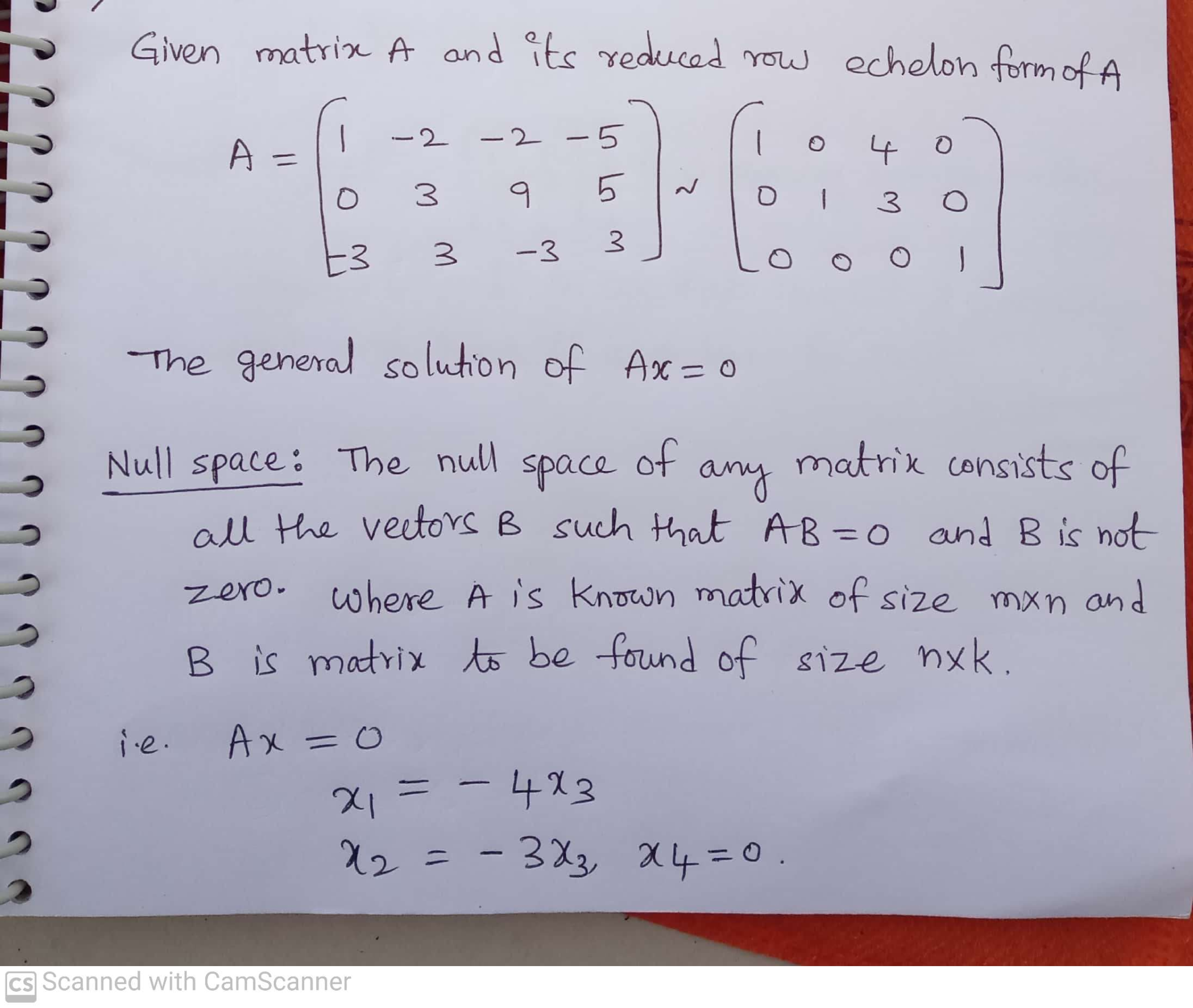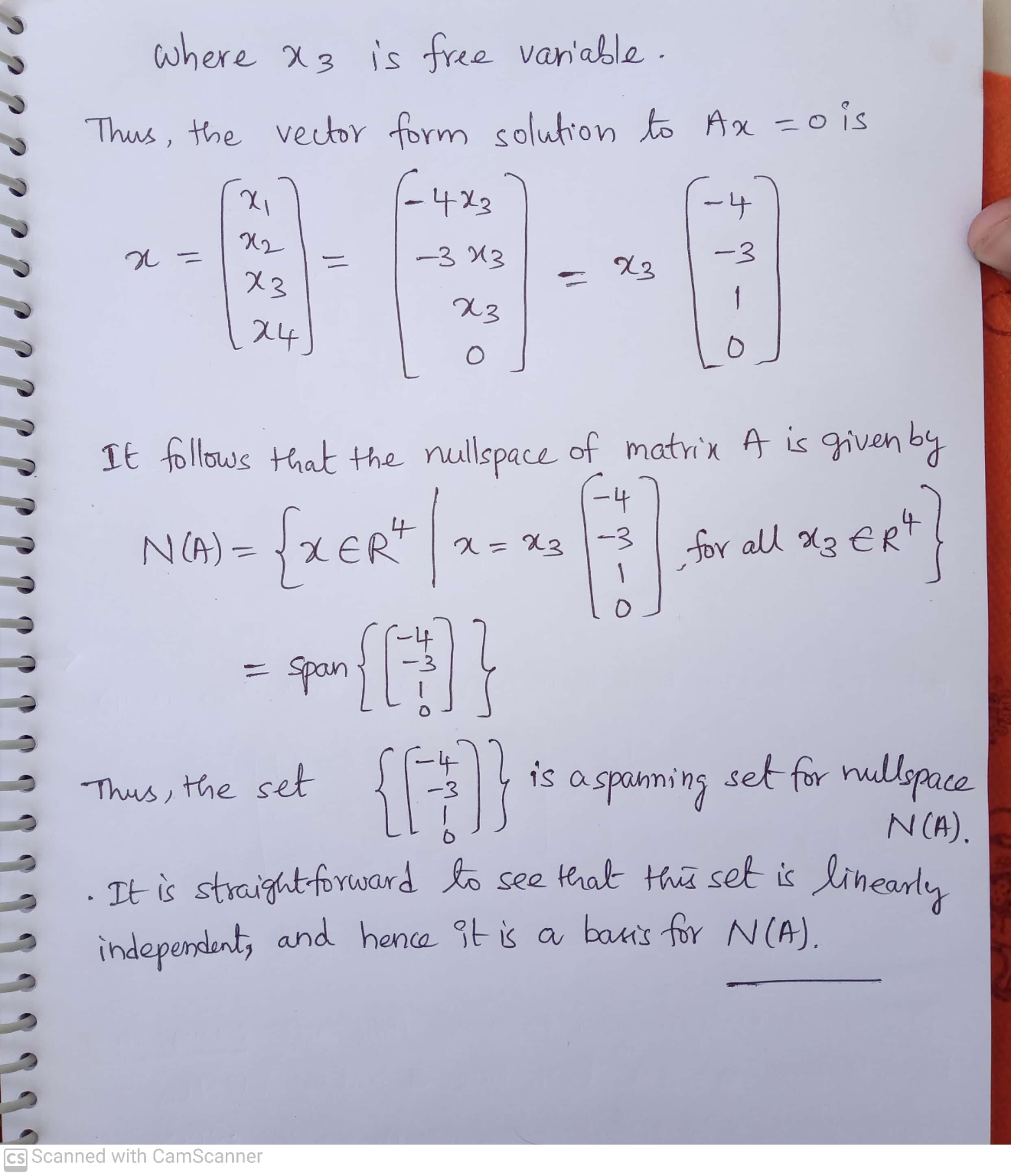##### Add Answer of: Consider the matrix A and the reduced row echelon form of A. 1 -2 -5 [1...
Similar Homework Help Questions
• ### Consider the matrix A and the reduced row echelon form of A. A 1 -2 -2...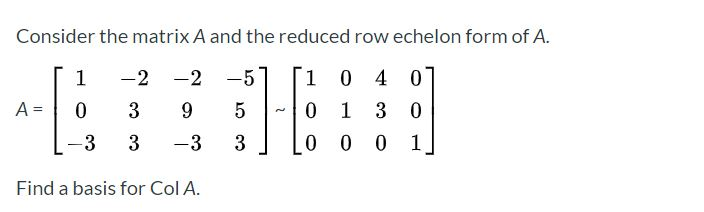Consider the matrix A and the reduced row echelon form of A. A 1 -2 -2 0 39 -3 3 -3 1 0 4 0 0 1 3 0 0 0 0 1 Find a basis for Col A.

• ### 1 1. The matrix A and it reduced echelon form B are given below. 1 -2...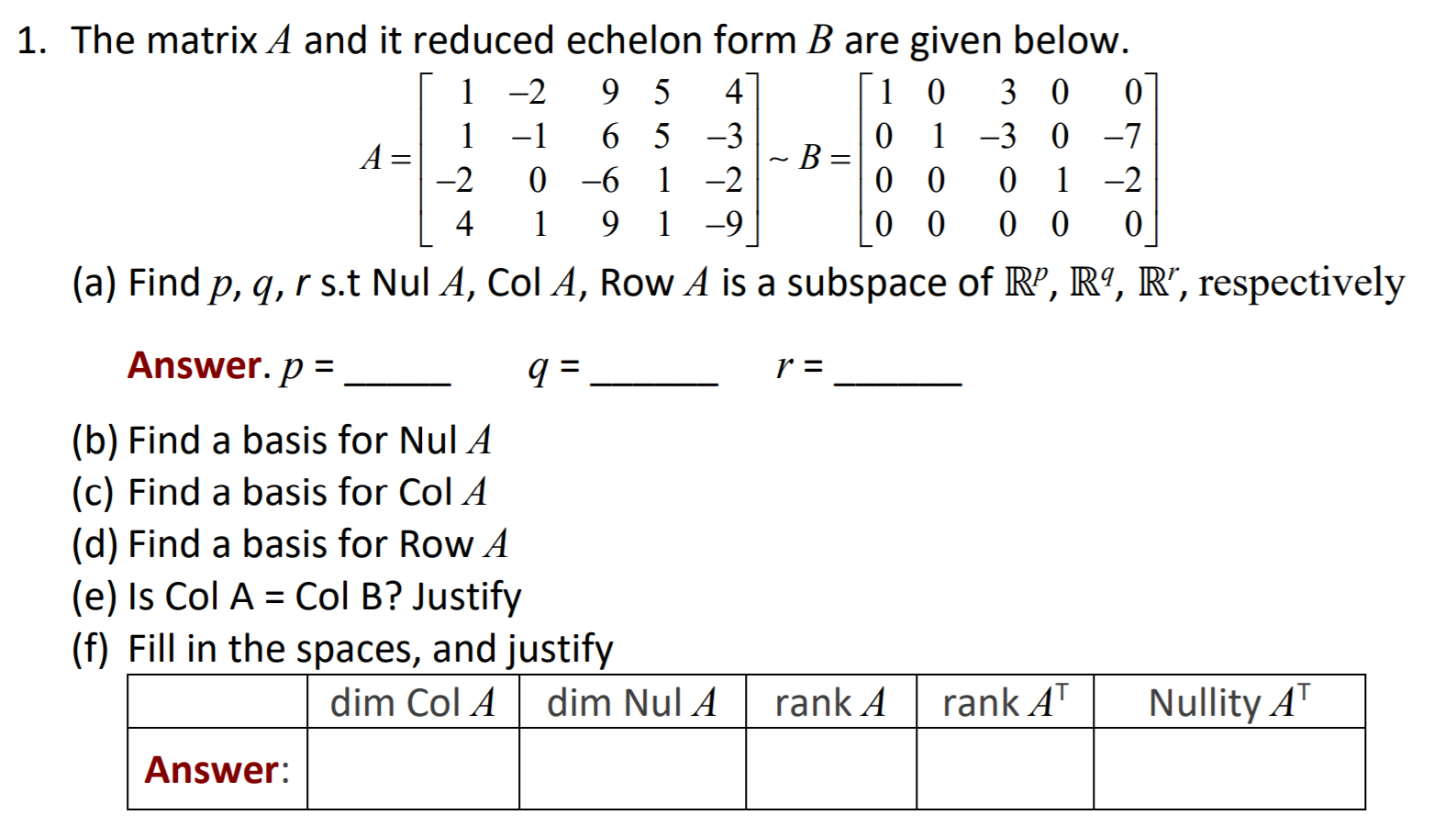1 1. The matrix A and it reduced echelon form B are given below. 1 -2 9 5 4 1 0 3 0 0 -1 6 5 -3 0 1 -3 0 -7 A= ~B= -2 0 -6 1 -2 0 0 1 -2 4 9 1 -9 0 0 0 0 0 (a) Find p, q, r s.t Nul A, Col A, Row A is a subspace of RP, R9, R”, respectively o 1 Answer. p = a =...

• ### 4 1 1. The matrix A and it reduced echelon form B are given below. 1-2...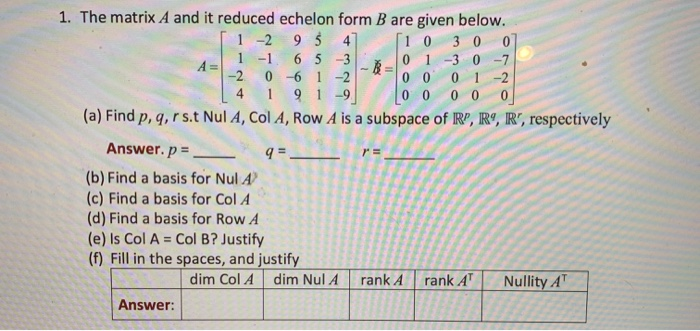4 1 1. The matrix A and it reduced echelon form B are given below. 1-2 95 4 10 3 0 0 1 -1 6 5 3 0 1 -3 0 -7 -2 0 -6 1 -2 0 0 0 1 -2 9 1-9 0 0 0 0 0 (a) Find p, q, r s t Nul A, Col A, Row A is a subspace of RP, R9, R', respectively Answer. p = 9= (b) Find a basis for Nul...

• ### 1. The matrix A and it reduced echelon form B are given below. 1-2 95 4...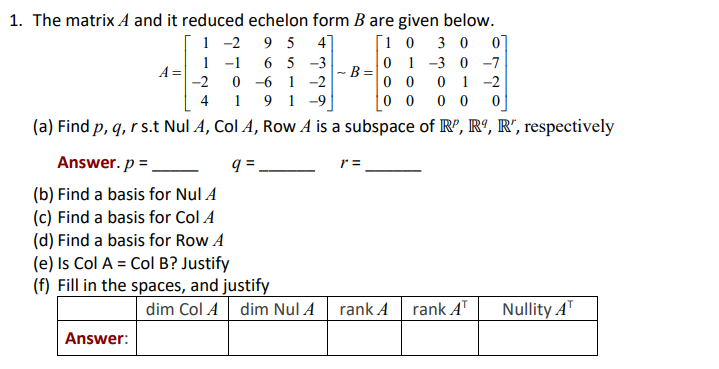1. The matrix A and it reduced echelon form B are given below. 1-2 95 4 [1 0 3 0 0 1 -1 6 5 -3 A= 0 1 -3 0 -7 -B= -2 0 -6 1 -2 0 0 0 1 -2 4 9 -9 0 0 0 0 0 (a) Find p, q, rs. Nul A, Col A, Row A is a subspace of R”, R9, R", respectively Answer.p = 9. r = (b) Find a basis for...

• ### 4. Give the row-echelon form and the reduced row-echelon form of the matrix: A = 11...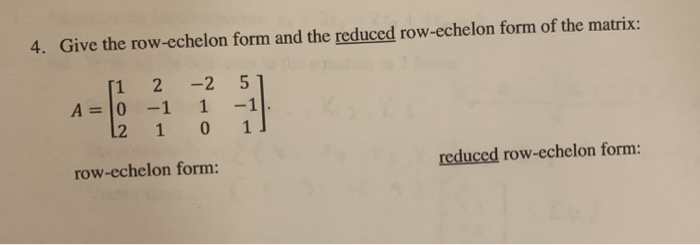4. Give the row-echelon form and the reduced row-echelon form of the matrix: A = 11 2 0 -1 12 1 -2 51 1 -1 0 1] row-echelon form: reduced row-echelon form:

• ### 4 1 1. The matrix A and it reduced echelon form B are given below. 1-2...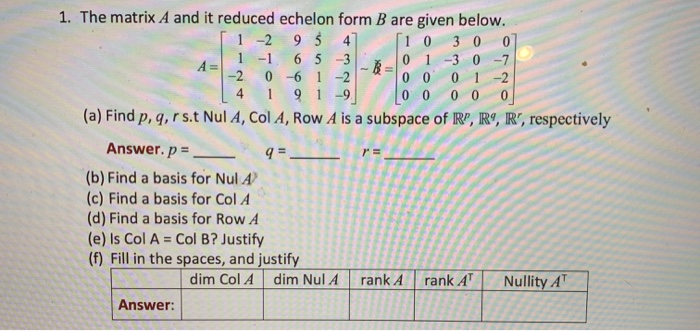4 1 1. The matrix A and it reduced echelon form B are given below. 1-2 95 4 10 3 0 0 1 -1 6 5 3 0 1 -3 0 -7 -2 0 -6 1 -2 0 0 0 1 -2 91-9 0 0 0 0 0 (a) Find p, q, rs. Nul A, Col A, Row A is a subspace of R", R9, R', respectively Answer. p = 9=- (b) Find a basis for Nul A (c) Find...

• ### Use row-reduction to put the following matrix to reduced row echelon form. 1 5 4 2...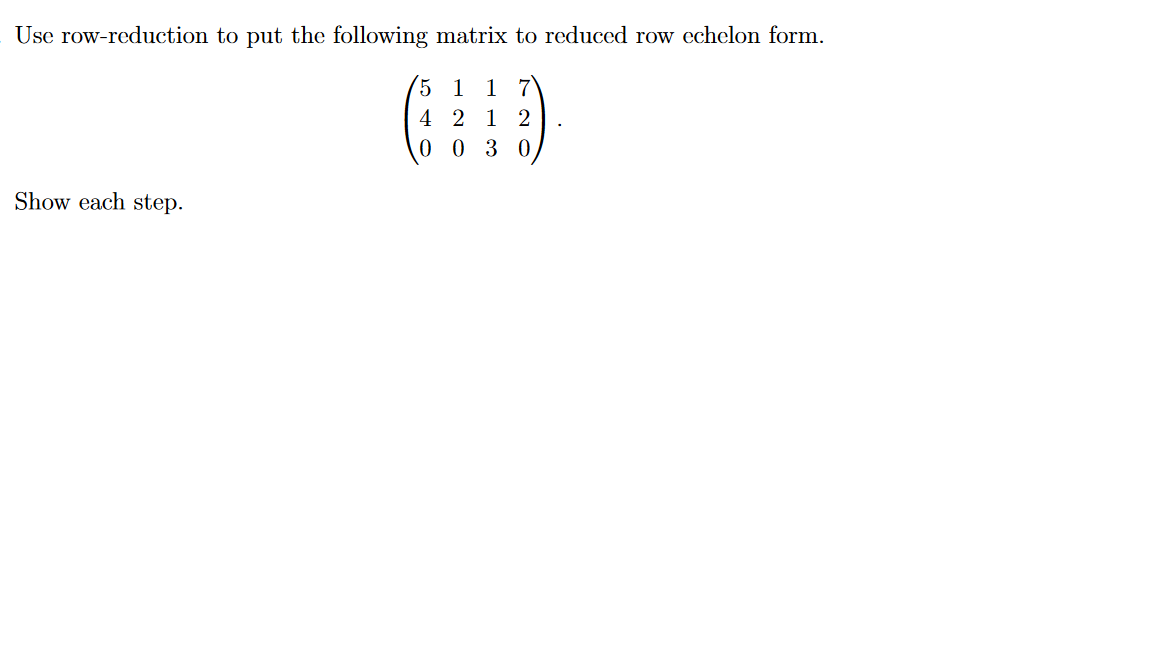Use row-reduction to put the following matrix to reduced row echelon form. 1 5 4 2 1 2 0 0 3 0 Show each step.

• ### 4. Consider the matrix 0- 3 1 -2 1 4 (a) Use Matlab to determine the reduced row echelon form of A (b) If v, V2, vs, v4...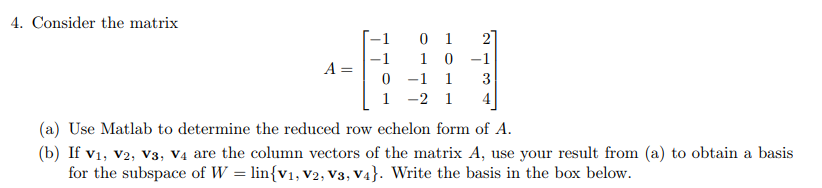4. Consider the matrix 0- 3 1 -2 1 4 (a) Use Matlab to determine the reduced row echelon form of A (b) If v, V2, vs, v4 are the column vectors of the matrix A, use your result from (a) to obtain a basis for the subspace of W-linsv1, V2, V3, V4. Write the basis in the box below 4. Consider the matrix 0- 3 1 -2 1 4 (a) Use Matlab to determine the reduced row echelon form...

• ### Question 3 Use row-reduction to put the following matrix to reduced row echelon form. 5 1...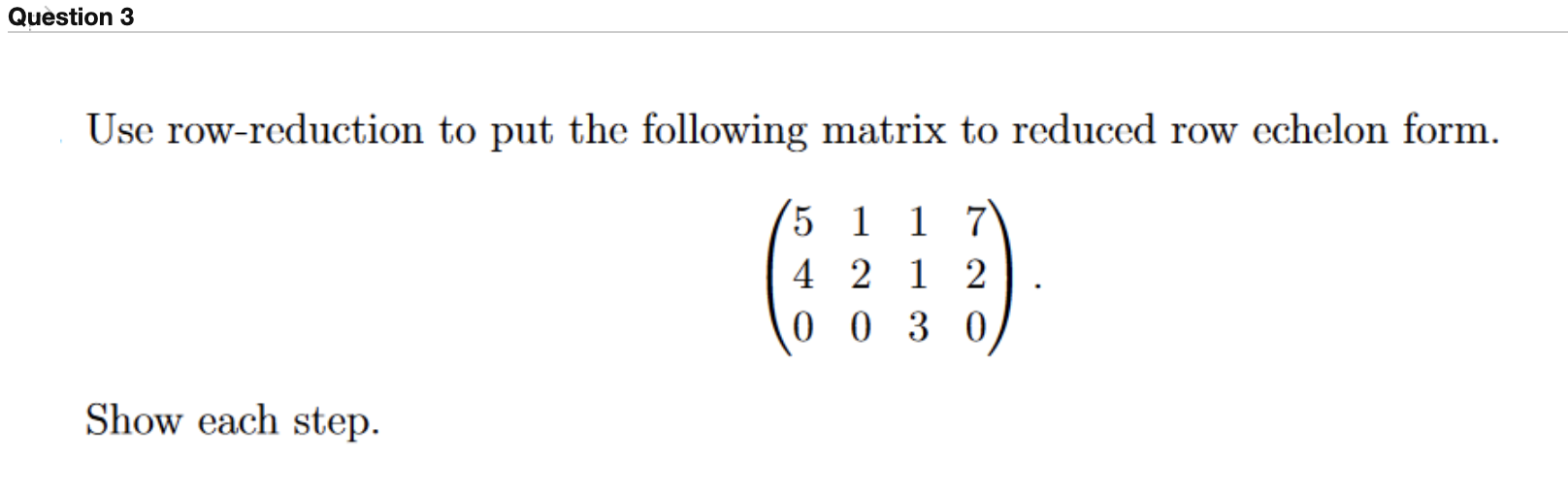Question 3 Use row-reduction to put the following matrix to reduced row echelon form. 5 1 1 7 4 2 1 2 0 0 3 0 Show each step.

• ### 3 3 -16 -2 -5 12 4 1-12 Find the reduced row echelon form of the...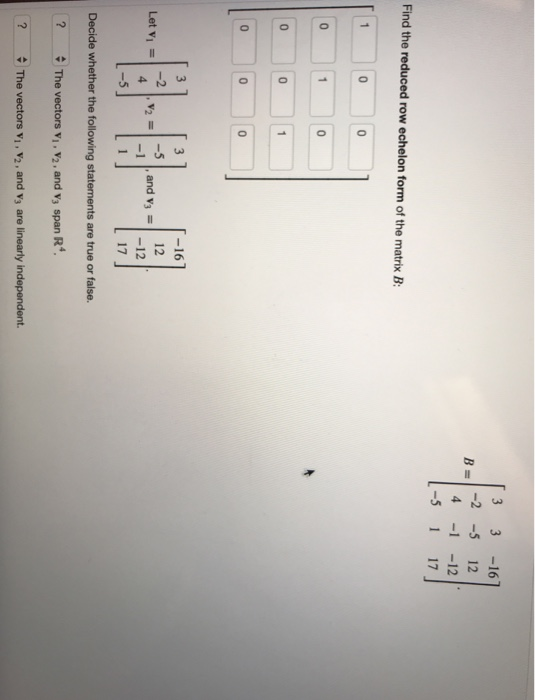3 3 -16 -2 -5 12 4 1-12 Find the reduced row echelon form of the matrix B 0 0 0 0 0 0 -16 12 -5 1, and v3 = 1-12 Let Vi 4 17 5 Decide whether the following statements are true or false. 2 The vectors vi, V2, and v span R. The vectors vi , V2 , and V3 are linearly independent. 3 3-16 В 1-2-5 4 -1 -12 Find the reduced row echelon form of...

Free Homework App